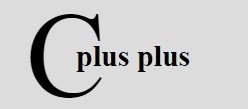# C++ Program Structure

« Previous Tutorial Next Tutorial »

Program Structure of a simple C++ program contains a header file, a function main(), then program code. Here is the program structure of a simple C++ program:

```header files
return_type main()
{
program_code_statements;
}```

Here is an example, showing the program structure of a simple C++ program:

```/* C++ Program Structure - This is a multi-line comment */
#include<iostream.h>          // header file. This is a single-line comment
void main()              // main function
{
clrscr();       // to clear the screen
cout<<"Hello, C++";      // prints Hello, C++
getch();           // holds output screen until user press a key
}```

### Example Explained

In the above program, the first line is a multi-line comments. Multi-line comment starts from /* and end with */. Then the second line and the third line, both are header files included in the program by using #include. The iostream.h header file is responsible for cout (standard output) and conio.h header file is responsible for the functions clrscr() (to clear the output screen) and then getch() (holds the output screen until user press a key). Now the fourth line contains main() function. Here void represents that the program will not return any value. And in last, between { and }, program_code_statements is present. In which, clrscr() function clears the output screen, cout is used to send the string "Hello, C++" on the output screen. In other words, cout<<"Hello, C++"; simply prints the string "Hello, C++" on the standard output. Now getch() is the function, responsible to hold the output screen until and unless, user press any key. You can see from the above program, we have included some short description after //, which indicates single-line comments. Anything after // becomes a single-line comment. Comments are totally ignored by the compiler. Here is the output of the above C++ program:

Let's take some more example to understand the structure of some simple C++ program:

```// My first simple C++ program
/* C++ Program Structure - This program
* prints Hello Compiler, I am C++ on
* the output screen. */

#include<iostream.h>
#include<conio.h>
void main()
{
clrscr();
cout<<"Hello Compiler, I am C++";
getch();
}```

Here is the sample output of the above C++ program:

Here is another type of simple C++ program

```/* C++ Program Structure - Example Program */

#include<iostream.h>
#include<conio.h>
void main()
{
clrscr();
char name;
cin>>name;
cout<<"Hello, "<<name;
getch();
}```

Here is the sample run of the above C++ program:

Here is one more type of C++ program using function.

```/* C++ Program Structure - Example Program */

#include<iostream.h>
#include<conio.h>
void msg(void);
void main()
{
clrscr();
char ch;
cout<<"Want to see the message ? (y/n).. ";
cin>>ch;
if(ch=='y' || ch=='Y')
{
msg();
}
cout<<"\n\nPress any key to exit...";
getch();
}
void msg(void)
{
cout<<"\nWelcome to codescracker.com";
cout<<"\n";
cout<<"This is C++ Program Structure Tutorial";
}```

Here are two sample run of this C++ program:

### More Examples

Here are some more type of C++ example programs listed, that you can go for:

C++ Online Test

« Previous Tutorial Next Tutorial »